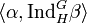# Maximum degree of irreducible representation of group is less than or equal to product of maximum degree of irreducible representation of subgroup and index of subgroup

## Statement

Suppose$G$ is a finite group,$H$ is a subgroup, and$K$ is a field whose characteristic does not divide the order of$G$ (we do not require$K$ to be a splitting field, though the splitting field case is of particular interest).

Then, the Maximum degree of irreducible representation (?) of$G$ over$K$ is less than or equal to the product:

(maximum degree of irreducible representation of$H$ over$K$) times (index of$H$ in$G$, i.e.,$[G:H]$)

## Proof

### Proof in characteristic zero

Given:$G$ is a finite group,$H$ is a subgroup, and$K$ is a field of characteristic zero.$H$ has index$d$ in$G$.$m$ is the maximum of the degrees of irreducible representations of$G$ over$K$.

To prove:$H$ has an irreducible representation over$K$ whose degree is at least$m/d$.

Proof:

Step no. Assertion/construction Facts used Given data used Previous steps used Explanation
1 Let$\alpha$ be the character of an irreducible representation of$G$ of degree$m$.$m$ is the maximum of degrees of irreducible representations of$G$.
2 Let$\beta$ be the character of an irreducible representation of$H$ arising as a subrepresentation of$\operatorname{Res}_H^G \alpha$.$H$ is a subgroup of the finite group$G$.
3 The inner product$\langle \operatorname{Res}_H^G \alpha, \beta \rangle_H$ is a positive integer, because$\beta$ is the character of a subrepresentation of the representation affording$\operatorname{Res}_H^G\alpha$. (Note: Over a splitting field, the inner product is precisely the multiplicity, but it may be bigger in general if$\beta$ is not absolutely irreducible). We need$K$ to have characteristic zero to make sense of positive integer.
4$\langle \operatorname{Res}_H^G \alpha, \beta \rangle_H = \langle \alpha, \operatorname{Ind}_H^G \beta \rangle$ Fact (2)
5$\langle \alpha, \operatorname{Ind}_H^G \beta \rangle$ is a positive integer, indicating that$\alpha$ is an irreducible constituent of$\operatorname{Ind}_H^G \beta$. Steps (3), (4)
6 The degree of$\alpha$, which is$m$, is less than or equal to the degree of$\operatorname{Ind}_H^G\beta$ Steps (1), (5)
7 The degree of$\operatorname{Ind}_H^G\beta$ is$d$ times the degree of$beta$$H$ has index$d$ in$G$. Definition of induced representation.
8 The degree of$beta$ is at least$m/d$ Steps (6), (7)